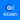# Lecture in Temperature and Kinetic Theory | Giancoli Physics

(Last Updated On: March 21, 2020)

### Lesson Objectives – the students should be able to:

• Convert a temperature given in degrees Fahrenheit to degrees Celsius and/or degrees Kelvin, and vice versa.
• State the factors that cause the volume of a solid or liquid to change or the length of a solid to change. Also, solve word problems and determine the final length or volume.
• Write the mathematical relationships that summarize Boyle’s law, Charles law, Gay Lussac’s law, and the ideal gas equation. Use these equations to solve word problems.
• State in your own words Avogadro’s hypothesis. State from memory the modern value of Avogadro’s number.
• State the postulates of the kinetic theory of gases.
• Rewrite the ideal gas equation in terms of motion of the molecules of an ideal gas.
• Explain what is meant by the term rms velocity.
• Explain what is meant by Van der Waal’s forces.
• Given a phase diagram for water, determine the range of temperature and pressure at which water is a solid, liquid, or gas. Describe what is meant by the triple point of water and point out the triple point on a phase diagram.
• Explain what is meant by sublimation and use a phase diagram to determine the range of temperatures and pressures for which the sublimation of water could occur.
• Explain why evaporation from a liquid is related to the temperature of the liquid and the average kinetic energy of the molecules of the liquid.
• Explain what is meant by vapor pressure and explain why vapor pressure is related to the temperature of the liquid and the boiling point of the liquid.
• Distinguish between relative humidity and absolute humidity and solve word problems related to relative humidity.
• Explain what is meant by diffusion and why diffusion is slower through a liquid than through a gas.
• Use Fick’s law to solve word problems related to gaseous diffusion.
• State Graham’s law of diffusion and use this law to determine the mass of a molecule of an unknown gas.

### Summary of Chapter 13

• All matter is made of atoms.
• Atomic and molecular masses are measured in atomic mass units, u.
• Temperature is a measure of how hot or cold something is, and is measured by thermometers.
• There are three temperature scales in use: Celsius, Fahrenheit, and Kelvin.
• When heated, a solid will get longer by a fraction given by the coefficient of linear expansion.
• The fractional change in volume of gases, liquids, and solids is given by the coefficient of volume expansion.
• Ideal gas law:
• One mole of a substance is the number of grams equal to the atomic or molecular mass.
• Each mole contains Avogadro’s number of atoms or molecules.
• The average kinetic energy of molecules in a gas is proportional to the temperature:
• Below the critical temperature, a gas can liquefy if the pressure is high enough.
• At the triple point, all three phases are in equilibrium.
• Evaporation occurs when the fastest moving molecules escape from the surface of a liquid.
• Saturated vapor pressure occurs when the two phases are in equilibrium.
• Relative humidity is the ratio of the actual vapor pressure to the saturated vapor pressure.
• Diffusion is the process whereby the concentration of a substance becomes uniform.

### Units of Chapter 13 – Keywords

• Atomic Theory of Matter
• Temperature and Thermometers
• Thermal Equilibrium and the Zeroth Law of Thermodynamics
• Thermal Expansion
• Thermal Stress
• The Gas Laws and Absolute Temperature
• The Ideal Gas Law
• Problem Solving with the Ideal Gas Law
• Ideal Gas Law in Terms of Molecules: Avogadro’s Number
• Kinetic Theory and the Molecular Interpretation of Temperature
• Distribution of Molecular Speeds
• Real Gases and Changes of Phase
• Vapor Pressure and Humidity
• Diffusion
• Brownian motion
• Thermometers
• mole

Help Me Makes a Difference!

 P inoyBIX educates thousands of reviewers/students a day in preparation for their board examinations. Also provides professionals with materials for their lectures and practice exams. Help me go forward with the same spirit. “Will you make a small gift today via GCASH?”+63 966 459 6474 Option 1 : \$1 USD Option 2 : \$3 USD Option 3 : \$5 USD Option 4 : \$10 USD Option 5 : \$25 USD Option 6 : \$50 USD Option 7 : \$100 USD Option 8 : Other Amount© 2014 PinoyBIX Engineering. © 2019 All Rights Reserved | How to Donate? |